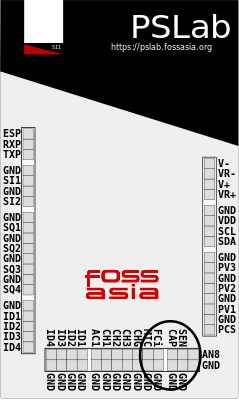# Measuring capacitor in PSLab and its Bugs

In this blog I will discuss about how we have measured capacitance in Pocket Science Lab and the  issues in capacitance measurement which was finally solved.

Measuring capacitance in PSLab device

To measure capacitance we need to go to the multimeter instrument from the instrument section of the PSLabFigure 1.  Showing Multimeter Tile

Capacitance in PSLab is measured by keeping the capacitor or the element of which capacitance is to be measured between the CAP and ground pin.Figure 2.  Showing CAP pins in PSLab

For measuring capacitance in PSLab we use a specific method in which we supply a constant current to the CAP pin and thus we charge the capacitor to the maximum level, the math involved in it is as follow:-

We know that

Q{charge stored} = C*V

Also

Q= I * time

Where

I=current (constant)

Thus the capacitance

C = Q / V

Therefore

C = I*time / V (measured). – (1)

Therefore we know the current supplied, we know the voltage measured and we have also set the time to charge the capacitor and thus we get the capacitance from equation (1).

Code implementation for measuring capacitance

This is the primary code for getting the data for measuring capacitance in which we pass the current range and the current time through which the data gets fetched from the device which is then further processed in another function in which we finally get the capacitance.

```public double[] getCapacitance(int currentRange, int chargeTime) { // time in uSec
try {
mPacketHandler.sendByte(mCommandsProto.COMMON);
mPacketHandler.sendByte(mCommandsProto.GET_CAPACITANCE);
mPacketHandler.sendByte(currentRange);
mPacketHandler.sendInt(chargeTime);
int VCode;
do VCode = mPacketHandler.getVoltageSummation();
while (VCode == -1);
double v = 3.3 * VCode / 4095;
mPacketHandler.getAcknowledgement();
double chargeCurrent = this.currents[currentRange];
double c = 0;
if (v != 0)
c = (chargeCurrent * chargeTime * 1e-6 / v - this.SOCKET_CAPACITANCE) / this.currentScalars[currentRange];
return new double[] {
v,
c
};
} catch (IOException | InterruptedException e) {
e.printStackTrace();
}
return null;```

In the above function we can clearly see how we send the bytes in the device by the sendByte function through which various functions are sending current, setting voltage, setting current range etc are done in the device and then we can see how the voltage measured is taken using the getVoltageSummition method (of packet Handler class) , how we get the current and finally using them in equation (1) we get the capacitance of the element.

The following implementation is taken from the PSLab desktop app where the same method is used to measure capacitance.

Bugs in measuring capacitance

The capacitance measurement although was working in the desktop app but had bugs in the android app. It could never read the correct value also everytime gave a null value for capacitance.Figure 3.  Showing null value for capacitance  PSLab

Solving the Bug 

After a deep research in the inside  the code of the capacitance measurement it was found that the error was caused while fetching the incorrect data from the device and processing it. The device gives a negative one value when there is any error in capacitance measurement  and that was being processed, thus the error was solved by introducing a do while loop as shown

``` do VCode = mPacketHandler.getVoltageSummation();
while (VCode == -1);```

And thus now only the correct data fetched is processed further and thus the bug was solved after which the capacitance measurement was correct.